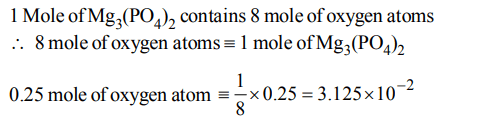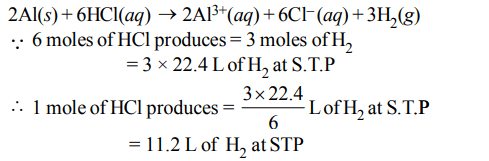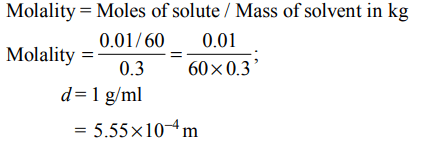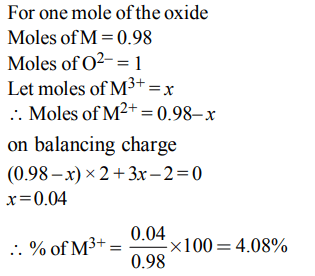## Some Basic Concepts of Chemistry Questions and Answers Part-7

1. If we consider that 1/6, in place of 1/12, mass of carbon atom is taken to be the relative atomic mass unit, the mass of one mole of the substance will
a) be a function of the molecular mass of the substance
b) remain unchanged
c) increase two fold
d) decrease twice

Explanation:2. How many moles of magnesium phosphate, $Mg_{3}\left(PO_{4}\right)_{2}$   will contain 0.25 mole of oxygen atoms?
a) $1.25 ×10^{-2}$
b) $2.5 ×10^{-2}$
c) 0.02
d) $3.125 ×10^{-2}$

Explanation:3. The density $\left(in g mL^{-1}\right)$  of a 3.60 M sulphuric acid solution that is 29% $H_{2}SO_{4}\left(molar mass = 98 g mol^{-1}\right)$      by mass will be
a) 1.45
b) 1.64
c) 1.88
d) 1.22

Explanation:4. In the reaction, $2AI\left(s\right)+6HCl\left(aq\right)\rightarrow2Al^{3+}\left(aq\right)+6CI^{-}\left(aq\right)+3H_{2}\left(g\right)$
a) 11.2 L $H_{2}\left(g\right)$  at STP is produced for every mole $HCI\left(aq\right)$  consumed
b) 6 L $HCl\left(aq\right)$  is consumed for every 3 L $H_{2}\left(g\right)$  produced
c) 33.6 L $H_{2}\left(g\right)$  is produced regardless of temperature and pressure for every mole Al that reacts
d) $67.2 H_{2}\left(g\right)$  at STP is produced for every mole Al that reacts.

Explanation:5. The molality of a urea solution in which 0.0100 g of urea, $\left[\left(NH_{2}\right)_{2}CO\right]$   is added to  $0.3000 dm^{3}$  of water at STP is :
a) $5.55*10^{-4}$  m
b) 33.3 m
c) $3.33*10^{-2}$  m
d) 0.555 m

Explanation:6. A gaseous hydrocarbon gives upon combustion 0.72 g of water and 3.08 g. of $CO_{2}$. The empirical formula of the hydrocarbon is :
a) $C_{2}H_{4}$
b) $C_{3}H_{4}$
c) $C_{6}H_{5}$
d) $C_{7}H_{8}$

Explanation:7. Experimentally it was found that a metal oxide has formula $M_{0.98}O$  . Metal M, present as  $M^{2+} and M^{3+}$   in its oxide. Fraction of the metal which exists as $M^{3+}$  would be :
a) 7.01%
b) 4.08%
c) 6.05%
d) 5.08%

Explanation:8. Consider a titration of potassium dichromate solution with acidified Mohr's salt solution using diphenylamine as indicator. The number of moles of Mohr's salt required per mole of dichromate is
a) 3
b) 4
c) 5
d) 6

Explanation:9. The correctly reported answer of addition of 29.4406, 3.2 and 2.25 will have significant figures
a) 3
b) 4
c) 2
d) 5